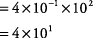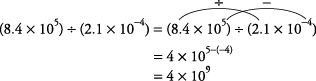## Scientific Notation

Very large or very small numbers are sometimes written in scientific notation. A number written in scientific notation is a number between 1 and 10 multiplied by a power of 10.

Express the following in scientific notation.

3,400,000

0.0000008

0.0047

274.3

3,400,000 written in scientific notation is 3.4 × 10 6. Simply place the decimal point to get a number between 1 and 10 and then count the digits to the right of the decimal to get the power of 10.moved six digits to the left

0.0000008 written in scientific notation is 8 × 10 –7.

Simply place the decimal point to get a number between 1 and 10 and then count the digits from the original decimal point to the new one.moved seven digits to the right

0.0047 written in scientific notation is 4.7 × 10 –3.

Simply place the decimal point to get a number between 1 and 10 and then count the digits from the original decimal point to the new one.moved three digits to the right

274.3 written in scientific notation is 2.743 × 10 2.

Simply place the decimal point to get a number between 1 and 10 and then count the digits from the original point to the new one.moved two digits to the left

Notice that numbers greater than 1 have positive exponents when expressed in scientific notation and numbers between 0 and 1 have negative exponents when expressed in scientific notation.

To multiply numbers in scientific notation, simply multiply the numbers that are between 1 and 10 together to get the first number and add the powers of ten to get the second number.

Multiply the following and express the answers in scientific notation.

(2 × 10 2)(3 × 10 4)

(6 × 10 5)(5 × 10 7)

(4 × 10 –4)(2 × 10 5)

(5 × 10 4)(9 × 10 2)

(2 × 10 2)(4 × 10 4)(5 × 10 6)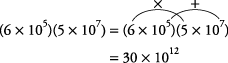This answer must be changed to scientific notation.

Change the 30 into its scientific notation form.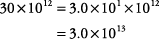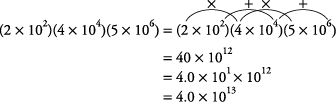To divide numbers in scientific notation, simply divide the numbers that are between 1 and 10 to get the first number and subtract the powers of ten to get the second number.

Divide the following and express the answers in scientific notation.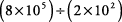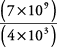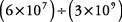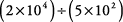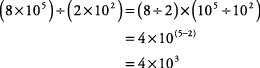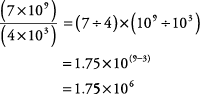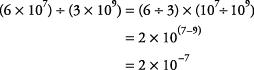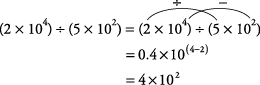Change 0.4 into its scientific notation form.